[MSE] iterated sin using Besselfunction 1st kindGottfriedUltimate FellowPosts: 900 Threads: 130 Joined: Aug 2007 10/30/2022, 12:38 PM (This post was last modified: 10/30/2022, 12:44 PM by Gottfried.) This looks much promising!           In Nov 21 someone proposed to base the computation of iterated sin() on a matrix of Bessel-functions, diagonalize and get iterated sin() as composition of  $a_1 \sin(x) + a_3 \sin(3x)+a_5 \sin(5x) +...$ See this link to MSE      Just fiddled a bit with it, using Pari/GP, here some code Code:dpow(D,h) = for(k=1,#D,D[k]=D[k]^h); matdiagonal(D)    \\ power of a diagonalmatrix, given only the vector of diagonal entries MPow(e)=tmpM * dpow(tmpD,e) * tmpW[,1]          \\ note: the diagonalization-matrices are to be recalculated if dimension parameter is changed                                                                         \\ in the OP in MSE the user needs only column 1 of tmpW sinit(h,x='x)=my(coeffs); coeffs=2^(h-1)*MPow(h-1);sum(k=1,#coeffs,coeffs[k]*sin((2*k-1)*x))                                         \\ for further experiments it may be more sensible to keep the "coeffs" as global to better access them when comparing etc dim=16     M=matrix(dim,dim,r,c,besselj(2*r-1,2*c-1));        \\ define Besselmatrix of size dim x dim     tmpM=mateigen(M);tmpW=tmpM^-1;tmpD=diag(tmpW*M*tmpM)  \\ do diagonalization \\ some tests [ w1=sinit(3,1) ; s1=sin(sin(sin(1)));s1 - w1] %372 = [    0.678430477361] [    0.678430477361] [1.29470815679 E-23] [x05=sinit(1/2,1);  x1=sinit(1/2,x05) ; s1=sin(1); s1-x1] %374 = [    0.908708429656] [    0.841470984758] [    0.841470984808] [5.00517837623 E-11] \\ ********* Increasing matrixsize *************** \\ Using dim=32     \\ \\ ... don't forget to recompute matrices! \\ [ w1=sinit(3,1) ; s1=sin(sin(sin(1)));s1 - w1] %378 = [    0.678430477361] [    0.678430477361] [1.75726847276 E-50] [x05=sinit(1/2,1);  x1=sinit(1/2,x05) ; s1=sin(1); s1-x1] %380 = [    0.908708429743] [    0.841470984808] [    0.841470984808] [9.73370904483 E-14] Looks very good, I think! Gottfried Gottfried Helms, KasselMphLeeLong Time FellowPosts: 374 Threads: 30 Joined: May 2013 12/17/2022, 02:34 PM Excuse me Gottfried... but reading the title I can't resist saying that if any function comes out of iterated sin using Bessel functions then you should call it the Hell function, or call the code Devil.Pari/GP.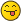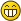MSE MphLee Mother Law $(\sigma+1)0=\sigma (\sigma+1)$ S Law $\bigcirc_f^{\lambda}\square_f^{\lambda^+}(g)=\square_g^{\lambda}\bigcirc_g^{\lambda^+}(f)$GottfriedUltimate FellowPosts: 900 Threads: 130 Joined: Aug 2007 12/17/2022, 03:06 PM (This post was last modified: 12/17/2022, 03:06 PM by Gottfried.) (12/17/2022, 02:34 PM)MphLee Wrote: Excuse me Gottfried... but reading the title I can't resist saying that if any function comes out of iterated sin using Bessel functions then you should call it the Hell function, or call the code Devil.Pari/GP.Hmm, perhaps getting famous & holy if one eventually finds the sin()-root or perhaps, better: root-sin() ?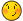(however, programming this in "Python", likely drives you out of paradise instead... isn't "Python" an objection-orientated language, btw.?) Gottfried Helms, KasselJmsNxnUltimate FellowPosts: 1,214 Threads: 126 Joined: Dec 2010 12/18/2022, 01:01 AM (This post was last modified: 12/18/2022, 05:34 AM by JmsNxn.) Hey, Gottfried. This is precisely the Fourier expansion of $\sin^{\circ 1/2}(x)$. It's well known this function is odd, and is also periodic. Thereby, when we expand the fourier expansion: $\sin^{\circ 1/2}(x) = \sum_{k=1}^\infty a_k \sin(kx)\\$ Where: $a_k = \frac{1}{2\pi}\int_{-\pi}^\pi \sin(kx)\sin^{\circ 1/2}(x)\,dx\\$ We can also deduce that $a_{2k} = 0$, because $\sin^{\circ 1/2}(x)(\pi - x) = \sin^{\circ 1/2}(x+\pi/2)$/// EDIT: SORRY I SCREWED UP THIS. I MEANT TO WRITE $\sin^{\circ 1/2}(x)(\pi - x) = \sin^{\circ 1/2}(x)$--which then eliminates even powers. I apoligize screwed that up a bit, lol. Which follows from basic trigonometric identities. Whereby we have a working: $\sin^{\circ 1/2}(x) = \sum_{k=0}^\infty a_{2k+1} \sin(2k+1)x\\$ That being said, I've never seen this connection to the Bessel function. Cool find!!!! It makes sense though because one of the formulae for the bessel function of the first kind is an integral of nested sinusoids over $[0,\pi]$; and expanding these $\sin^{\circ 1/2}(x)$, will result in something very similar. I also think, this provides a cool interchange of variables. Write: $\sin^{\circ 1/2}(x) = \frac{1}{\sqrt{\pi}}\int_0^\infty \frac{\vartheta(t,x)}{\sqrt{t}}\,dt\\$ Where: $\vartheta(t,x) = \sum_{n=0}^\infty \sin^{\circ n+1}(x) \frac{(-t)^n}{n!}\\$ Then; then we can interchange these integrals, so that: $a_{k}(1/2) = \frac{1}{\sqrt{\pi}} \int_0^\infty \sum_{n=0}^\infty a_{k}(n+1)\frac{(-t)^nt^{-1/2}}{n!} \,dt\\$ where: $a_k(s) = \frac{1}{2\pi} \int_{-\pi}^\pi \sin^{\circ s}(x) \sin(kx)\,dx\\$ I've been aware of this formula for a long time, but I never saw the relation to Bessel before! Also, note--I've never been able to prove this expansion of $\sin^{\circ 1/2}(x)$ is analytic. I only managed to show that it was  $C^{\infty}$ on $(-\pi,\pi)$. But I absolutely suspect it is analytic here. Maybe with closed forms of $a_k$ we'll have better luck!!!! I'd be really interested to see if this Fourier transform converges analytically, or only $C^\infty$. The sin iteration tends to be an odd ball out trying to get analycity out of. And showing uniform convergence of Fourier series in the complex plane is always a lot harder than it sounds (I think they've awarded like 4 fields medals for tiny step theorems on the convergence of Fourier series! lol!)GottfriedUltimate FellowPosts: 900 Threads: 130 Joined: Aug 2007 12/18/2022, 01:36 AM Hi James -  happy to see you saw this remark on the sinning-with-Bessel-functions. I've been already composing a PM to you, but cannot concentrate on one subject for enough time long... perhaps better next year. Since the main point of the PM should have been the pointing to this connection, it seems I can release now that half-ready draft :-)   Well, putting some comment here & there, perhaps a bit more, but I think more coherent stuff next year ... :-) Kind regards - Gottfried Gottfried Helms, KasselJmsNxnUltimate FellowPosts: 1,214 Threads: 126 Joined: Dec 2010 12/18/2022, 01:54 AM (This post was last modified: 12/18/2022, 06:44 AM by JmsNxn.) I'm excited, Gottfried. I have a fair amount of time over the holidays, and then come January 10th, I'm homefree for 2-3 months. And I plan to work a lot on some of the ideas I've been mulling over--specifically cementing a lot of iteration theory using the Mellin transform! I'm busting out a lot of old notes which hand waived the crucial details, and rigorously justify them much more. Look forward to working with you!I'm excited to deep dive into the "Matrix method is the same as the integral method." Regards, James EDIT! Just a quick proof to show that the $1,3,5,7,...$ sequence in Gottfried's original post is nothing special. $f(x) \,\,\text{is C^2 in}\,\,(-\pi,\pi)\\$ Additionally, assume that: $\int_{-\pi}^\pi |f(x)|^2\,dx < \infty\\$ This is true for any iterate of $\sin$. Now: $f(\pi - x) = f(x)\\$ What I think is more important here, is that $f(x-\pi) = -f(x)$. Which is what happens for every iterate of $\sin$. So let's work with the identity: $f(x+\pi) = - f(x)$. Therefore: $2\pi a_k = \int_{-\pi}^\pi f(x)sin(kx)\,dx = \int_0^\pi \left(f(x)\sin(kx)-(-1)^kf(x)\sin(kx)\right)\,dx$ Even values of $k$ are zero; odd values non-zero. Additionally, since: $\sin^{\circ n}(-x) = -\sin^{\circ n}(x)\\$ We know: $\int_{-\pi}^\pi f(x) \cos(kx)\,dx =0\\$ This is because this integrand is odd, across a symmetrical domain. Whereby, $f(-x)\cos(-kx) = -f(x)\cos(kx)$; and since we are integrating about zero over the same domain, the negative domain cancels the positive. And so, when we call on the traditional Fourier series: $f(x) = f(0) + \sum_{k=1}^\infty c_k \cos(kx) + a_k \sin(kx)\\$ We know, ABSOLUTELY, that $c_k = 0$ and $a_{2k} = 0$ and $f(0) = 0$. So that: $f(x) = \sum_{k=0}^\infty a_{2k+1} \sin(2k+1)x\\$ Is the default expansion. So the fact the MSE question pulled out this expansion is very much non-surprising, lol. We want to use this to show that the integral transform coefficients I generate, are the same as the Bessel function coefficients this MSE question pulled out. I'd bet 50\$ they're the same expansion. But the matrix approach looks much more promising. Definitely, the Bessel matrices are quicker and more accurate--but they are the same thing mathematically as my integrals... Anyway... I'm dead for tonight....MphLeeLong Time FellowPosts: 374 Threads: 30 Joined: May 2013 12/18/2022, 09:32 AM (12/17/2022, 03:06 PM)Gottfried Wrote: Hmm, perhaps getting famous & holy if one eventually finds the sin()-root or perhaps, better: root-sin() ? Holy is too much... maybe the Pope will appoint you a Sanctae Romanae Ecclesiae Transfinite Cardinalis. MSE MphLee Mother Law $(\sigma+1)0=\sigma (\sigma+1)$ S Law $\bigcirc_f^{\lambda}\square_f^{\lambda^+}(g)=\square_g^{\lambda}\bigcirc_g^{\lambda^+}(f)$GottfriedUltimate FellowPosts: 900 Threads: 130 Joined: Aug 2007 12/18/2022, 02:06 PM (12/18/2022, 09:32 AM)MphLee Wrote: (12/17/2022, 03:06 PM)Gottfried Wrote: Hmm, perhaps getting famous & holy if one eventually finds the sin()-root or perhaps, better: root-sin() ? Holy is too much... maybe the Pope will appoint you a Sanctae Romanae Ecclesiae Transfinite Cardinalis. Hmm, perhaps a sweet new pseudonym... "St. RET. Cardinalis" ... let's see.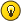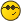Gottfried Helms, Kassel « Next Oldest | Next Newest »

 Possibly Related Threads… Thread Author Replies Views Last Post 4 hypothesis about iterated functions Shanghai46 11 458 04/22/2023, 08:22 PM Last Post: Shanghai46 Question about the properties of iterated functions Shanghai46 9 372 04/21/2023, 09:07 PM Last Post: Shanghai46 Iterated function convergence Daniel 1 367 12/18/2022, 01:40 AM Last Post: JmsNxn Uniqueness of fractionally iterated functions Daniel 7 1,477 07/05/2022, 01:21 AM Last Post: JmsNxnIterated Hyperbolic Sine and Iterated Natural Logarithm Catullus 2 951 06/11/2022, 11:58 AM Last Post: tommy1729 [MSE] Help on a special kind of functional equation. MphLee 4 2,333 06/14/2021, 09:52 PM Last Post: JmsNxn Generalized Kneser superfunction trick (the iterated limit definition) MphLee 25 15,018 05/26/2021, 11:55 PM Last Post: MphLee iterated derivation Xorter 0 2,751 06/09/2019, 09:43 PM Last Post: Xorter 1st iterated derivatives and the tetration of 0 Xorter 0 3,614 05/12/2018, 12:34 PM Last Post: Xorter Iterated nand Xorter 2 8,103 03/27/2017, 06:51 PM Last Post: Xorter

Users browsing this thread: 1 Guest(s)